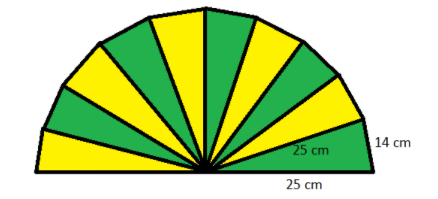A hand fan is made by stitching 10 equal size triangular strips of two different types of paper as shown in the figure. The dimensions of equal strips are 25cm, 25cm, and 14 cm. Find the area of each type of paper needed to make the hand fan.$\left( a \right)280{\text{ c}}{{\text{m}}^2}$$\left( b \right)420{\text{ c}}{{\text{m}}^2}$$\left( c \right)840{\text{ c}}{{\text{m}}^2}$$\left( d \right)384{\text{ c}}{{\text{m}}^2}$Verified
146.7k+ views
Hint: In this particular question use the concept that if all the sides of the triangle are given then the area (A) of the triangle is given as, $A = \sqrt {s\left( {s - a} \right)\left( {s - b} \right)\left( {s - c} \right)}$, so use this concept to reach the solution of the question.

Given data:
The sides of the triangle are 25cm, 25cm, and 14cm, as shown in the given figure.
Now we have to find out the area of each type of paper needed to make the hand fan.
Now as we see that there are two types of paper used in the given figure each type has 5 similar triangles.
As there are a total 10 triangles in a hand fan.
So the area $\left( {{A_1}} \right)$ of one type of paper = area $\left( {{A_2}} \right)$ of other types of paper = 5 times the area (A) of any one triangle.
Let, a = 25cm, b = 25cm, and c = 14cm.
Now as we know that if all the sides of the triangle are given then the area (A) of the triangle is given as,
$\Rightarrow A = \sqrt {s\left( {s - a} \right)\left( {s - b} \right)\left( {s - c} \right)}$................. (1), where s is the half of the perimeter and a, b and c are the length of the sides of the triangle.
Now as we know that the perimeter is the sum of all the sides.
So, the perimeter of the triangle = a + b + c = 25 + 25 + 14 = 64cm.
So, $s = \dfrac{{a + b + c}}{2} = \dfrac{{64}}{2} = 32$ cm.
Now substitute all the values in equation (1) we have,
$\Rightarrow A = \sqrt {32\left( {32 - 25} \right)\left( {32 - 25} \right)\left( {32 - 14} \right)}$
Now simplify this we have,
$\Rightarrow A = \sqrt {32\left( 7 \right)\left( 7 \right)\left( {18} \right)}$
$\Rightarrow A = \sqrt {{7^2}\left( {16} \right)\left( {36} \right)}$
$\Rightarrow A = \sqrt {\left( {{7^2}} \right)\left( {{4^2}} \right)\left( {{6^2}} \right)}$
$\Rightarrow A = 7\left( 4 \right)\left( 6 \right) = 168$ Sq. cm.
So the area of each type of paper needed to make the hand fan is 5 times the above calculated area.
$\Rightarrow {A_1} = {A_2} = 5\left( A \right) = 5\left( {168} \right) = 840{\text{ c}}{{\text{m}}^2}$
Hence option (c) is the correct answer.

Note:Whenever we face such types of questions the key concept we have to remember is that the perimeter of any shape is the sum of all the sides, so the perimeter of triangle is the sum of all the sides, so calculate it, so s is equal to half of the perimeter so calculate it then simply substitute the values in the area formula as above and simplify we will get the required answer.